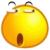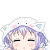## javascript问题：rgb语法书写问题

为什么80%的码农都做不了架构师？->>>document.body.style.backgroundColor = 'rgb('+ Math.floor(Math.random() * 256)+','+Math.floor(Math.random() * 256)+','+Math.floor(Math.random() * 256)+')';

'rgb('+ ……的一长串字串连接，是怎么连接起来的？

谢谢。

0```'rgb('+ Math.floor(Math.random() * 256)+','+Math.floor(Math.random() * 256)+','+Math.floor(Math.random() * 256)+')';

'rgb(' + n1 + ',' + n2 + ',' + n3 + ')'

// 拼接之后就是字符串
'rgb(n1,n2,n3)'```

0```'a' + 'b' // 'ab'
'a' + 3   // 'a3'```